MORE IN Structural Analysis - 2
MU Civil Engineering (Semester 5)
Structural Analysis - 2
December 2014
Total marks: --
Total time: --
INSTRUCTIONS
(1) Assume appropriate data and state your reasons
(2) Marks are given to the right of every question
(3) Draw neat diagrams wherever necessary

1 (a) Determine the degree of static and kinematic in determinacy of the following structures.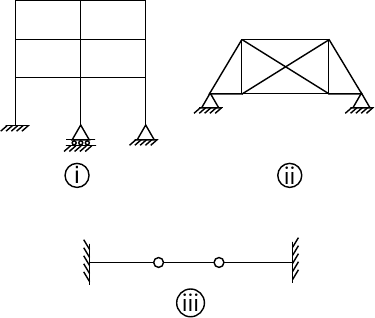6 M
1 (b) Develop the flexibility and stiffness matrix for prismatic member AB with reference to the co-ordinates shown in fig.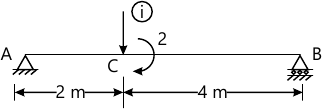10 M
Define the following terms.
1 (c) (i) Carry over factor.
1 M
1 (c) (ii) Distribution factor.
1 M
1 (c) (iii) Relative stiffness and modified stiffness.
2 M

2 (a) Analyse the beam by flexibility method and draw BMD.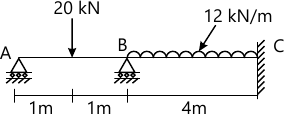10 M
2 (b) Analyse the pin joined plane frame shown in fig. By flexibility method.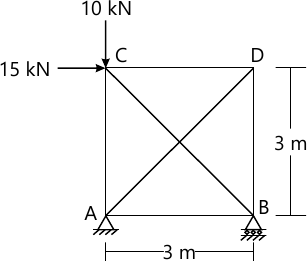10 M

3 Analyse the frame and draw BMD by using flexibility method.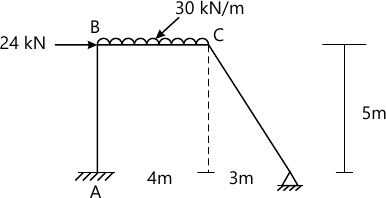20 M

4 (a) Analyse frame by slope defection method and draw B.M.D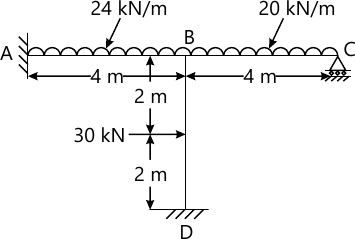12 M
4 (b) Analyse the beam by three moment theorem and Draw B.M.D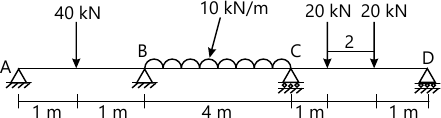8 M

5 (a) Analyse the frame by moment distribution method.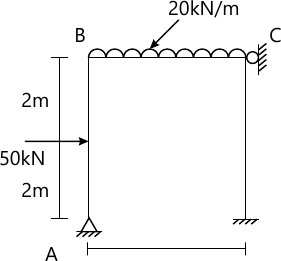12 M
5 (b) Determine the plastic moment capacity for the bean as shown in fig. Lake load factor 1.5.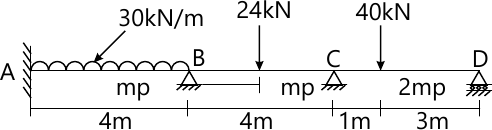8 M

6 (a) Analyse the frame by stiffness method and dran B.M.D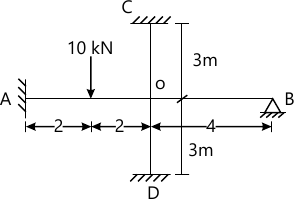12 M
6 (b) A two hinged parabolic arch of span-40m and rise 10 m carries uniformly distributed 10 and 30k/m on right half span find the reaction at the sup ports and draw B.M.D
8 M

More question papers from Structural Analysis - 2